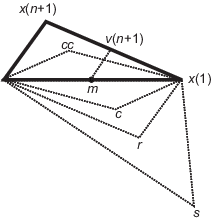## 优化非线性函数

### 求一元函数的最小值

```x = -1:.01:2; y = humps(x); plot(x,y) xlabel('x') ylabel('humps(x)') grid on````x = fminbnd(@humps,0.3,1)`
```x = 0.6370 ```

```options = optimset('Display','iter'); x = fminbnd(@humps,0.3,1,options)```
``` Func-count x f(x) Procedure 1 0.567376 12.9098 initial 2 0.732624 13.7746 golden 3 0.465248 25.1714 golden 4 0.644416 11.2693 parabolic 5 0.6413 11.2583 parabolic 6 0.637618 11.2529 parabolic 7 0.636985 11.2528 parabolic 8 0.637019 11.2528 parabolic 9 0.637052 11.2528 parabolic Optimization terminated: the current x satisfies the termination criteria using OPTIONS.TolX of 1.000000e-04 ```
```x = 0.6370 ```

### 求多元函数的最小值

`fminsearch` 函数与 `fminbnd` 类似，不同之处在于前者处理多变量函数。请指定起始向量 x0，而非起始区间。`fminsearch` 尝试返回一个向量 x，该向量是数学函数在此起始向量附近的局部最小值。

```function b = three_var(v) x = v(1); y = v(2); z = v(3); b = x.^2 + 2.5*sin(y) - z^2*x^2*y^2;```

```v = [-0.6,-1.2,0.135]; a = fminsearch(@three_var,v) a = 0.0000 -1.5708 0.1803```

### 求函数最大值

`fminbnd``fminsearch` 求解器尝试求目标函数的最小值。如果您有最大化问题，即以下形式的问题：

`$\underset{x}{\mathrm{max}}f\left(x\right),$`

```[x fval] = fminbnd(@(x)-tan(cos(x)),3,8) x = 6.2832 fval = -1.5574```

### `fminsearch` 算法

`fminsearch` 使用 Lagarias 等人的著作  中所述的 Nelder-Mead 单纯形算法。此算法对 n 维向量 x 使用 n + 1 个点组成的单纯形。此算法首先向 x0 添加各分量 x0(i) 的 5%，以围绕初始估计值 x0 生成一个单纯形。然后，该算法使用上述 n 个向量作为单纯形的除 x0 之外的元素。（如果 x0(i) = 0，则算法使用 0.00025 作为分量 i）。然后，此算法按照以下过程反复修改单纯形。

`fminsearch` 迭代输出方式中的关键字在相应的步骤说明后以粗体形式显示。

1. 用 x(i) 表示当前单纯形中的点列表 i = 1,...,n + 1。

2. 按最小函数值 f(x(1)) 到最大函数值 f(x(n + 1)) 的顺序对单纯形中的点进行排序。在迭代的每个步骤中，此算法都会放弃当前的最差点 x(n + 1) 并接受单纯形中的另一个点。[或者在下面的步骤 7 中，此算法会更改值在 f(x(1)) 上方的所有 n 个点。]

3. 生成反射

 r = 2m – x(n + 1)， (1)

其中

 m = Σx(i)/n, i = 1...n， (2)

并计算 f(r)。

4. 如果 f(x(1)) ≤ f(r) < f(x(n))，则接受 r 并终止此迭代。反射

5. 如果 f(r) < f(x(1))，则计算延伸点 s

 s = m + 2(m – x(n + 1))， (3)

并计算 f(s)。

1. 如果 f(s) < f(r)，接受 s 并终止迭代。扩展

2. 否则，接受 r 并终止迭代。反射

6. 如果 f(r) ≥ f(x(n))，则在 m 和 x(n + 1) 或 r（取目标函数值较低者）之间执行收缩

1. 如果 f(r) < f(x(n + 1))（即 r 优于 x(n + 1)），则计算

 c = m + (r – m)/2 (4)

并计算 f(c)。如果 f(c) < f(r)，则接受 c 并终止迭代。外收缩

否则，继续执行步骤 7（收缩）。

2. 如果 f(r) ≥ f(x(n + 1))，则计算

 cc = m + (x(n + 1) – m)/2 (5)

并计算 f(cc)。如果 f(cc) < f(x(n + 1))，则接受 cc 并终止迭代。内收缩

否则，继续执行步骤 7（收缩）。

7. 计算 n 点

 v(i) = x(1) + (x(i) – x(1))/2 (6)

并计算 f(v(i))，i = 2,...,n + 1。下一迭代中的单纯形为 x(1), v(2),...,v(n + 1)。收缩### 参考

 Lagarias, J. C., J. A. Reeds, M. H. Wright, and P. E. Wright. “Convergence Properties of the Nelder-Mead Simplex Method in Low Dimensions.” SIAM Journal of Optimization, Vol. 9, Number 1, 1998, pp. 112–147.